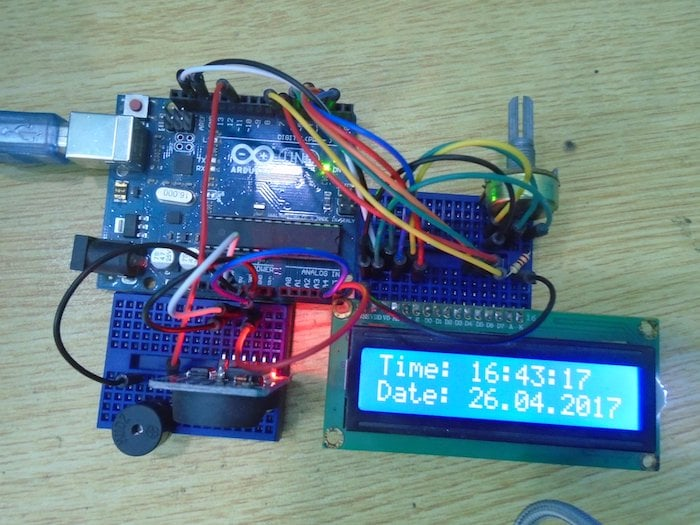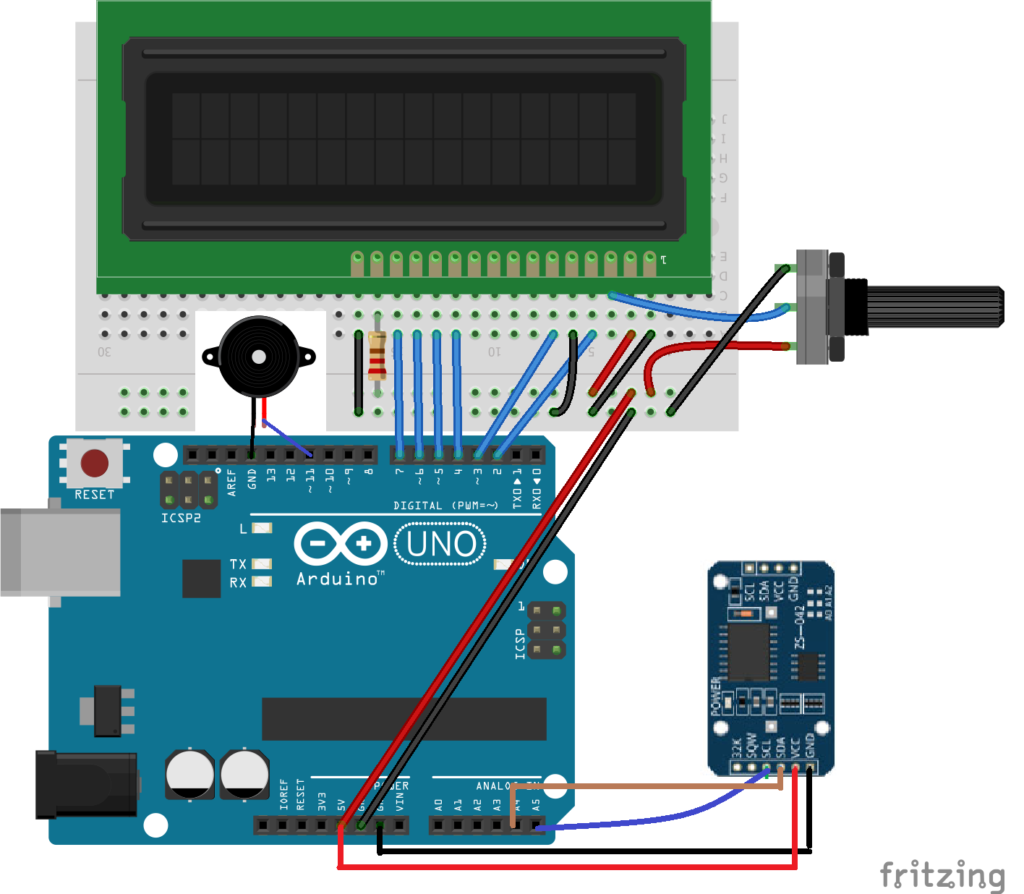Make your own Arduino alarm clock using a DS3231 real-time clock module.In this project, we are going to make an Arduino alarm clock using the DS3231 real time clock module. This module is very cheap and works through I2C communication, which makes it easy to use with the microcontrollers. We will get the time using this module and will make the buzzer beep after comparing the current time with the alarm time. This module also tells us the temperature.

### How Does the Arduino Alarm Clock Work?

The DS3231 real time clock module keeps track of the time even when the module is not powered. It has a built-in 3V battery, which keeps updating the time. We will get the time and date from the RTC module using the library functions and then we will compare this time with the alarm time that we have set in the code.

If the current time matches with the alarm time, then the buzzer will start to beep. The beeping time in our code is 2 minutes, but you can increase it as much you want. The time and date will also be displayed on the LCD.

### Circuit Diagram

Connect the LCD to the Arduino as follows:

• Pin 1 on the LCD to ground on the Arduino.
• Pin 2 on the LCD to 5V on the Arduino.
• Pin 3 on the LCD to the middle pin on the 10K potentiometer.
• Pin 4 on the LCD to digital pin 2 on the Arduino.
• Pin 5 on the LCD to the ground of Arduino. This will put the LCD in read mode.
• Pin 6 on the LCD to the pin 3 of Arduino.
• Connect the data pins (D4-D7) to the pins 4, 5, 6, 7 on the Arduino.
• Pin 15 to the 5V pin on the Arduino through the 220-ohm resistor. This is the positive pin of the backlight.
• Pin 16 to ground on the Arduino. This is the negative pin of the backlight.

Then connect the DS3231 real time clock module to the Arduino as follows:

• GND on the DS3231 to GND on the Arduino
• VCC on the DS3231 to the 5V pin on the Arduino
• SCL on the DS3231 to A5 on the Arduino
• SDA on the DS3231 to A4 on the Arduino
Finally, connect the positive of the buzzer to pin 11 on the Arduino and the negative the of buzzer to GND on the Arduino.### Arduino Alarm Clock Code

```#include <DS3231.h>

#include <Wire.h>

#include <LiquidCrystal.h>

LiquidCrystal lcd(2, 3, 4, 5, 6, 7);

DS3231  rtc(SDA, SCL);

Time  t;

#define buz 11

int Hor;

int Min;

int Sec;

void setup()

{

Wire.begin();

rtc.begin();

Serial.begin(9600);

pinMode(buz, OUTPUT);

lcd.begin(16,2);

lcd.setCursor(0,0);

lcd.print("DIYHacking.com");

lcd.setCursor(0,1);

lcd.print("Arduino Alarm ");

// The following lines can be uncommented to set the date and time

//rtc.setDOW(WEDNESDAY);     // Set Day-of-Week to SUNDAY

//rtc.setTime(12, 0, 0);     // Set the time to 12:00:00 (24hr format)

//rtc.setDate(1, 1, 2014);   // Set the date to January 1st, 2014

delay(2000);

}

void loop()

{

t = rtc.getTime();

Hor = t.hour;

Min = t.min;

Sec = t.sec;

lcd.setCursor(0,0);

lcd.print("Time: ");

lcd.print(rtc.getTimeStr());

lcd.setCursor(0,1);

lcd.print("Date: ");

lcd.print(rtc.getDateStr());

if( Hor == 11 &&  (Min == 32 || Min == 33)) //Comparing the current time with the Alarm time

{

Buzzer();

Buzzer();

lcd.clear();

lcd.print("Alarm ON");

lcd.setCursor(0,1);

lcd.print("Alarming");

Buzzer();

Buzzer();

}

delay(1000);

}

void Buzzer()

{

digitalWrite(buz,HIGH);

delay(500);

digitalWrite(buz, LOW);

delay(500);

}```

### Code Explanation

First of all, we included the libraries for the DS3231 real time clock and the LCD. The wire library is for the I2C communication between the DS3231 and the Arduino. The DS3231 real time clock communicates with the Arduino through the I2C communication, so we have included the wire library.
```
#include <DS3231.h>

#include <Wire.h>

#include <LiquidCrystal.h>```

After that, we initialized the variables that we are going to use in this code.

```LiquidCrystal lcd(2, 3, 4, 5, 6, 7);

DS3231  rtc(SDA, SCL);

Time  t;

#define buz 11

int Hor;

int Min;

int Sec;```

The below lines are commented in the code. These lines are for setting the date and time for the RTC module. You won’t have to set the date and time every time you use it, because the RTC module has a battery that keeps working even when the module is not powered.

```//rtc.setDOW(WEDNESDAY);     // Set Day-of-Week to SUNDAY

//rtc.setTime(12, 0, 0);     // Set the time to 12:00:00 (24hr format)

//rtc.setDate(1, 1, 2014);   // Set the date to January 1st, 2014```

In the loop function, we get the time from the module and store it in the variables. We will compare these variables later with the alarm time we want to set.

```t = rtc.getTime();

Hor = t.hour;

Min = t.min;

Sec = t.sec;```

After that, we print the time and date on the LCD screen.

```lcd.setCursor(0,0);

lcd.print("Time: ");

lcd.print(rtc.getTimeStr());

lcd.setCursor(0,1);

lcd.print("Date: ");

lcd.print(rtc.getDateStr());```

Then we compare the current time with the alarm time, and if the time matches, the buzzer will start to beep. The buzzer will keep on beeping for 2 minutes, but you can increase or decrease the beeping time as much you want.

```if( Hor == 11 &&  (Min == 32 || Min == 33)) //Comparing the current time with the Alarm time

{

Buzzer();

Buzzer();

lcd.clear();

lcd.print("Alarm ON");

lcd.setCursor(0,1);

lcd.print("Alarming");

Buzzer();

Buzzer();

}```